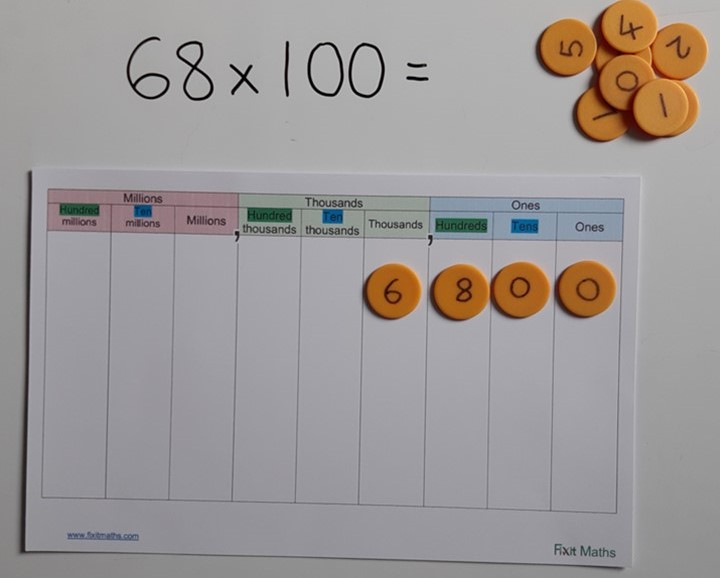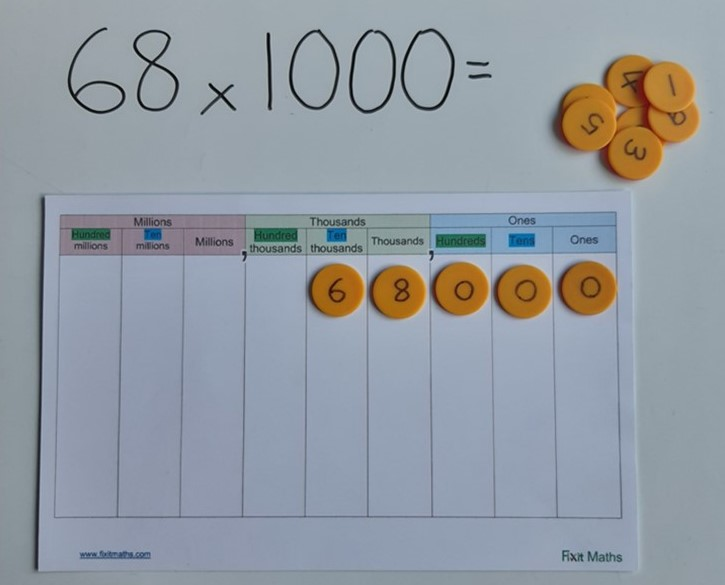## Multiplying by 100 or 1000

When a number is multiplied by 100, each part of that number becomes 100 times larger. If we break this down, it is the same as becoming 10 times larger, then 10 times larger again. So a one becomes a ten and then a hundred, a ten becomes a hundred then a thousand etc. This can easily be seen using Dienes.Using a place value grid, we can see that the digits move two places to the left as they are multiplied by 100, representing how the value of each has become 100 times larger. Two place holders are needed to show that there are now no tens or ones.When multiplying by 1000, each digit becomes 1000 times larger, so a one cube now becomes a 1000. On a place value grid, we can see that the digits move 3 places to the left.The number of zeroes in the number we are multiplying by (10, 100 or 1000) reminds us how many places to move on the grid.

Download an activity pack of games to reinforce multiplying or dividing by 10, 100 or 1000
Click here to generate worksheet to practise multiplying and dividing by 10, 100 & 1000.